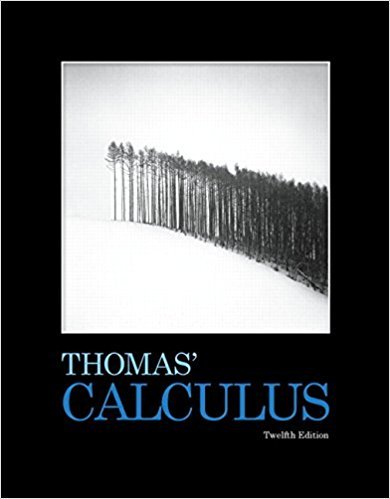×
×

# Solutions for Chapter 5: Integration## Full solutions for Thomas' Calculus | 12th Edition

ISBN: 9780321587992Solutions for Chapter 5: Integration

Solutions for Chapter 5
4 5 0 337 Reviews
26
1
##### ISBN: 9780321587992

Since 86 problems in chapter 5: Integration have been answered, more than 8634 students have viewed full step-by-step solutions from this chapter. This expansive textbook survival guide covers the following chapters and their solutions. Chapter 5: Integration includes 86 full step-by-step solutions. This textbook survival guide was created for the textbook: Thomas' Calculus, edition: 12. Thomas' Calculus was written by and is associated to the ISBN: 9780321587992.

Key Calculus Terms and definitions covered in this textbook
• Absolute minimum

A value ƒ(c) is an absolute minimum value of ƒ if ƒ(c) ? ƒ(x)for all x in the domain of ƒ.

• Arc length formula

The length of an arc in a circle of radius r intercepted by a central angle of u radians is s = r u.

• Center

The central point in a circle, ellipse, hyperbola, or sphere

• Empty set

A set with no elements

• Least-squares line

See Linear regression line.

• Linear inequality in two variables x and y

An inequality that can be written in one of the following forms: y 6 mx + b, y … mx + b, y 7 mx + b, or y Ú mx + b with m Z 0

• Matrix, m x n

A rectangular array of m rows and n columns of real numbers

• Multiplicative inverse of a matrix

See Inverse of a matrix

• nth root

See Principal nth root

• Obtuse triangle

A triangle in which one angle is greater than 90°.

• Order of magnitude (of n)

log n.

• Parameter

See Parametric equations.

• Quotient of complex numbers

a + bi c + di = ac + bd c2 + d2 + bc - ad c2 + d2 i

• Quotient polynomial

See Division algorithm for polynomials.

• Random numbers

Numbers that can be used by researchers to simulate randomness in scientific studies (they are usually obtained from lengthy tables of decimal digits that have been generated by verifiably random natural phenomena).

• Solution of a system in two variables

An ordered pair of real numbers that satisfies all of the equations or inequalities in the system

• Supply curve

p = ƒ(x), where x represents production and p represents price

• Weighted mean

A mean calculated in such a way that some elements of the data set have higher weights (that is, are counted more strongly in determining the mean) than others.

• Xmax

The x-value of the right side of the viewing window,.

• Ymax

The y-value of the top of the viewing window.

×